# Exercise 11.3 Mensuration- NCERT Solutions Class 8

Go back to  'Mensuration'

## Chapter 11 Ex.11.3 Question 1

There are two cuboidal boxes as shown in the adjoining figure. Which box requires the lesser amount of material to make?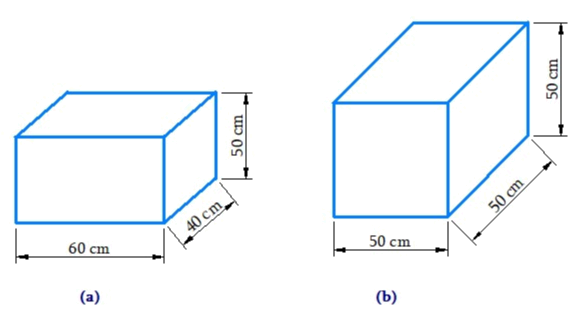### Solution

What is Known?

Dimensions of the cuboidal boxes.

What is unknown?

Surface area of the given figure

Reasoning:

The amount of material requires to make boxes will be equal to their respective surface area.

Steps:

Total surface area of the cuboid lateral surface $$=$$ area of curved surface $$+ 2\; \times$$ area of base

Total surface area of the cub $$= 6{(l)^2}$$

Total surface area of cuboid in figure

\begin{align} &= \! (hl \! + \! hb \! + \! hl \! + \! hb) \! + \! 2(lb)\\&= \! 2(hl \! + \! lb \! + \! hb)\\&= \! 2(60 \! \times \! 50 \! \times \! + \! 40 \! \times \! 50 \! + \! 60 \! \times \! 40)\,\rm{cm^2}\\&= \! 2(3000 \! + \! 2000 \! + \! 2400)\,\rm{cm^2}\\&= \! 14800\,\rm{m^2} \end{align}

Total surface area of cube in figure (b)

\begin{align} &= 6 \times {(l)^2}\\& = 6 \times {(50)^2}\\& = 6 \times 2500\\&= 15000\,\rm{cm^2}\end{align}

Thus, the cuboid box (a) requires lesser amount of material.

## Chapter 11 Ex.11.3 Question 2

A suitcase with measures $$80\,\rm{ cm} × 48\, \rm{cm} × 24\,\rm{ cm}$$ is to be covered with a tarpaulin cloth. How many meters of tarpaulin of width $$96\,\rm{cm}$$ is required to cover $$100$$ such suitcases?

### Solution

What is Known?

Number of suitcases to be covered and dimensions of each suitcase.

What is unknown?

Required length of tarpaulin cloth cover suitcase

Reasoning:

Find the surface area of a suitcase.

Steps:

Let the length of the tarpaulin cloth $$= l \,\rm{cm}$$

Total surface of the suitcase

\begin{align} &= 2[(80\! \times\! 48) \!+\! (48\! \times\! 24) \!+\!(24 \!\times \!80)]\,{\rm{cm}^2}\\&= 2[3840 + 1152 + 1920]\,{\rm{c}}{{\rm{m}}^2}\\ &= 13824 \,\rm{m^2} \end{align}

Total surface area of $$100$$ suitcases

\begin{align} &= 100 \times 13824\,{{\rm{m}}^2}\\&= 1382400\,\,{\rm{cm}^2}\end{align}

Required Tarpaulin cloth

$= {\rm{Length\! \times\! Breadth}}$

$$1382400\,{\rm{c}}{{\rm{m}}^2} = l \times 96\,{\rm{cm}}$$

\begin{align}l& = \frac{{1382400}}{{96}}\,\,{\rm{cm}}\\&= 14400\,\,{\rm{cm}}\\l& = 144\,\rm{m}\end{align}

Thus, $$144\;\rm{ m}$$ of tarpaulin cloth is required to cover $$100$$ suitcases.

## Chapter 11 Ex.11.3 Question 3

Find the side of a cube whose surface area is $$600\,\rm{cm^2}$$.

### Solution

What is Known?

Surface area of cube.

What is unknown?

Length of the cube.

Steps:

Let the length of each side of cube be $$l.$$

Given that, surface area of cube $$=60\,{\rm{cm}^2}$$

Surface area of cube $$= 6 \times {\rm{(side)}^2}$$

\begin{align}600\,\,{\rm{cm}^2} &= 6 \times {(l)^2}\\{l^2} &= 100\,\,{\rm{c}}{{\rm{m}}^2}\\l &= 10\,{\rm{cm}} \end{align}

Thus, the side of the cube is $$10\,\rm{cm.}$$

## Chapter 11 Ex.11.3 Question 4

Rukhsar painted the outside of the cabinet of measure $$1\,\rm{m} \times 2\, \rm{m} \times \,1.5\, \rm{m.}$$ How much surface area did she cover if she painted all except the bottom of the cabinet?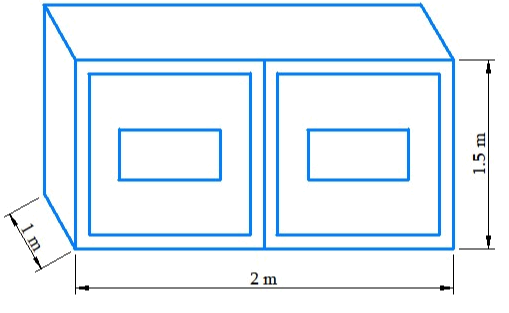### Solution

What is Known?

Dimensions of the cuboid.

What is unknown?

Surface area of cuboid that was palindrome.

Reasoning:

Surface area of bottom of the cuboid will be subtracted from the total surface area of the cabinet.

Steps:

Length ($$l$$) of the cabinet $$=\,2\,\rm{m}$$

Breadth ($$b$$) of the cabinet $$=1\,\rm{m}$$

Height ($$n$$) of the cabinet $$= 1.5\,\rm{m}$$

Area of the bottom of cabinet $$= (l \times b)$$

Total Surface area of the cabinet $$= 2(l \times b + b \times h + n \times l)$$

Area of the cabinet that was painted $$=$$ (Total surface area of  the cabinet) $$-$$ (Area of bottom of the cabinet)

\begin{align} &= 2(l \times b + b \times h + h \times l) - l \times b\\&= 2(b \times h + h \times l) + l \times b\\ &= [2 \times (1 \times 1.5 + 1.5 \times 2) + 2 \times 1]\,{{\rm{m}}^2}\\&= [2 \times 4.5 + 2]\,{{\rm{m}}^2}\\&= 11\,{{\rm{m}}^2} \end{align}

Hence, the required area $$= 11 \,\rm{m}^2$$

## Chapter 11 Ex.11.3 Question 5

Daniel is painting the walls and ceiling of a cuboidal hall with length, breadth and height of $$15\,\rm{ m}, 10\,\rm{ m}$$ and $$7\,\rm{ m}$$ respectively. From each can of paint $$100\,\rm{ m^2}$$ of area is painted. How many cans of paint will she need to paint the room?

### Solution

What is Known?

Shape of the hall and its length, breadth and height.

What is unknown?

Number of cans of paint required to paint the room.

Reasoning: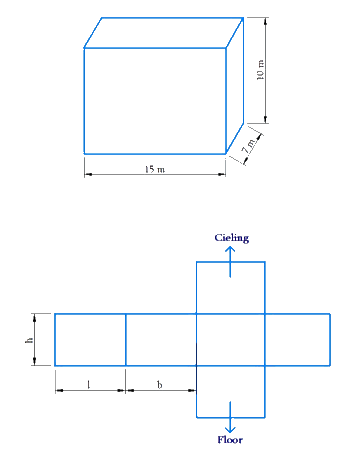The side of the hall makes the lateral surface area of cuboid. The total surface area of the cuboid will be the sum of lateral surface area of four walls and sum of the area of the ceiling and area of the floor.

Steps:

Length of the hall $$l =15\,\rm{m}$$

Breadth of the hall $$b=10\,\rm{m}$$

Height of the hall $$n=7\,\rm{m}$$

Lateral surface area of four walls

\begin{align}&= (h \times l + h \times b + h \times l + h \times b)\\& = 2(7 \times 15 + 7 \times 10)\,{{\text{m}}^2}\\&= 2(h \times l + h \times b)\\&= 2(105 + 70)\,{{\rm{m}}^2}\\&= 350\,{{\rm{m}}^2} \end{align}

Area of the ceiling $$= l \times b$$

$= 15 \times 10 = 150\,{{\rm{m}}^2}$

Area of the hall to be painted $$=$$ Lateral surface area of walls $$+$$ Area of the ceiling.

\begin{align} &= 350 \,\rm{m^2} + 150{m^2}\\ &= 500\,\rm{m^2} \end{align}

It is given that from each can $$100\,\rm{m^2}$$ area can be painted .

$$\therefore$$ Number of cans required to paint an area of \begin{align} 500\,\rm{m^2} = \frac{{500}}{{100}} = 5 \,cans \end{align}

Thus, $$5$$ cans are required to paint the walls and the ceiling of the cuboidal hall.

## Chapter 11 Ex.11.3 Question 6

Describe how the two figures at the right are alike and how they are different. Which box has larger lateral surface area?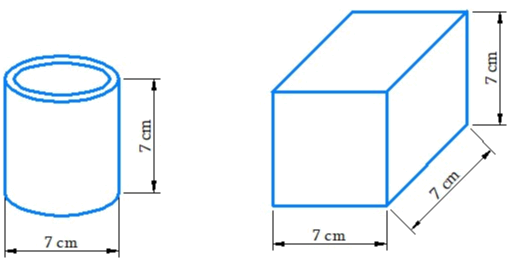### Solution

What is Known?

Shape and their respective dimension.

What is unknown?

Lateral surface area.

Reasoning: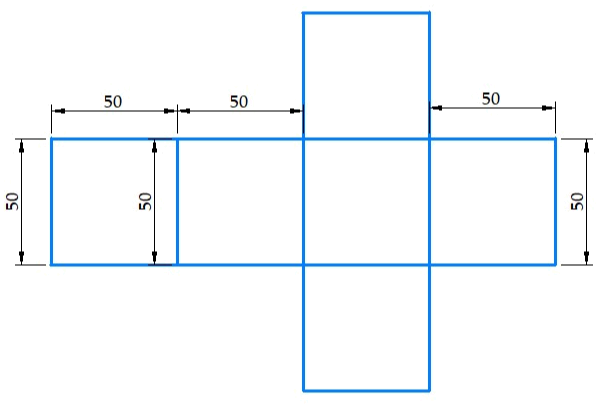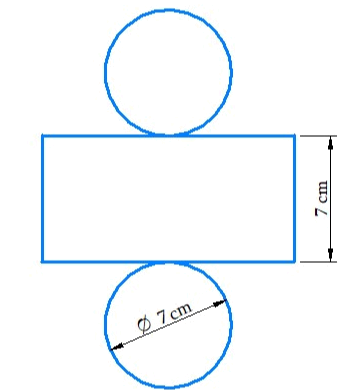Length of rectangular strip will be equal to the circumference of the circle. Visually, all the faces of a cube and square are equal in shape. This makes length, height and width of a cube equal, so area of each of the face will be equal.

Steps:

Similarly, both the figures are alike in respect of their same height.

The difference between the two figures is that one is cylinder and other is a cube.

Length of one side of cube $$\,(l) = 7\, \rm{cm}$$

Height of one side of cube $$\,(h) = 7\, \rm{cm}$$

Width of one side of cube $$\,(b) = 7\, \rm{cm}$$

Lateral surface area of the cube

\begin{align}&= (h\! \times\! l \!+\! h\! \times\! b\!+\! h\! \times \!l \!+\! h\! \times\! b)\\ &= (l\! \times\! l \!+\! l \!\times\! l\! +\! l \times l \!+ \! l \times l)\\&\qquad \because \{ l \!= \!h \!=\! b\} \\&= 4{l^2}\\ &= 4 \times {(7)^2}\\&= 196\,{{\rm{m}}^2} \end{align}

Height of the cylinder $$h = 7\,\rm{cm}$$

\begin{align}r = \frac{7}{2}\,\rm{cm} = 3.5\,\rm{cm} \end{align}

Lateral surface area of the cylinder

\begin{align}&= 2\pi rh\\&= 2 \times \frac{{22}}{7} \times 3.5 \times 7\\&=154\, \rm{m^2} \end{align}

Hence, the cube has larger lateral surface area.

## Chapter 11 Ex.11.3 Question 7

A closed cylindrical tank of radius $$7 \,\rm{m}$$ and height $$3\,\rm{ m}$$ is made from a sheet of metal. How much sheet of metal is required?

### Solution

What is Known?

Radius and height of cylindrical tank.

What is Unknown?

Metal sheet required for cylindrical tank.

Reasoning: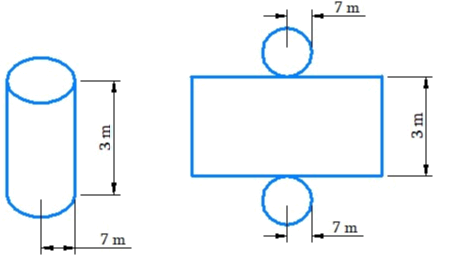The length of rectangular sheet will be equal to the circumference of the circle. Visually total surface of the cylinder will be equal to sum of lateral surface area and area of two circles.

Steps:

Height of the cylindrical tank $$(h) = 3\,\rm{cm}$$

Radius of the cylindrical $$(r) = 7\,\rm{cm}$$

Lateral surface area of the cylinder

\begin{align}&=2\pi rh \\&= 2 \times \frac{{22}}{7} \times 7 \times 3 \\&= 132\, \rm{m^2} \qquad \dots (\rm{1}) \end{align}

Area of circle

\begin{align}&= \pi {r^2} \\&= 2 \times \frac{{22}}{7} \times 7{\rm{m}} \times 7{\rm{m}} \\&=154\,{{\rm{m}}^2} \qquad \dots (\rm{2}) \end{align}

Total surface area of cylinder = Lateral surface area of the cylinder + Area of two circles
\begin{align}& = 2\pi rh + 2\pi {r^2}\\& = 132\, \rm{m^2} + 308 \,\rm{m^2}\\& \;\text{(putting values from (1) and (2))}\\ &= 440 \,\rm{m^2}\end{align}

Thus, $$440\,\rm{m^2}$$ sheet is required.

## Chapter 11 Ex.11.3 Question 8

The lateral surface area of a hollow cylinder is $$4224\, \rm{cm^2}$$ .It is cut along its height and formed a rectangular sheet of width $$33\,\rm{ cm.}$$ Find the perimeter of rectangular sheet?

### Solution

What is Known?

A hollow cylinder is cut along its height to from a rectangular sheet. The lateral surface of the cylinder is given.

What is unknown?

Perimeter of the rectangular sheet.

Reasoning:

The length of rectangular sheet will be equal to the circumference of the circle. Lateral surface area of the cylinder will be equal to the area of rectangular sheet.

Steps:

Lateral surface area of a hollow cylinder $$=4224\,\rm{m^2}$$

Width of rectangular sheet $$= 33\,\rm{cm}$$

Let length of the rectangular sheet $$=l$$

Area of cylinder = Area of rectangular sheet

\begin{align}4224\,\rm{m^2} &= 33 \rm{cm} \times l\\l &= \frac{{4224}}{{33}}\,\rm{cm}\\&= 128\,\rm{cm} \end{align}

Thus, the length of the rectangular sheet is $$128 \,\rm{cm.}$$

Perimeter of the rectangular sheet

\begin{align} &=2 \times ({\rm{length}} \times {\rm{width}})\\&= 2 \times (128\,{\rm{cm}} \times 33\,{\rm{cm}})\\&= 2 \times 161\,{\rm{cm}}\\&= 322\,{\rm{cm}}\end{align}

Thus, the perimeter of rectangular sheet is $$322\,\rm{ cm.}$$

## Chapter 11 Ex.11.3 Question 9

A road roller takes $$750$$ complete revolutions to move once over to level a road. Find the area of the road if the diameter of a road roller is $$84\,\rm{cm}$$ and length is $$1\,\rm{ m.}$$

### Solution

What is Known?

Diameter of the road roller and length is given.

What is unknown?

Reasoning:

In one revolution, the roller will cover an area equal to its lateral surface area.

Steps:

\begin{align}r = \frac{{84}}{2}\,\rm{cm} = 42\,\rm{ cm} \end{align}

Length of the road roller, $$h = 1\,\rm{m} = 100\, \rm{cm}$$

In $$1$$ revolution, area of the road covered

\begin{align} &= 2\pi rh \\&= 2 \times \frac{{22}}{7} \times 42 \times 100\\&= 26400\,{\rm{c}}{{\rm{m}}^2} = 2.64\,{{\rm{m}}^2} \end{align}

In $$750$$ revolutions area of the road covered

\begin{align} &= 750 \times 2.64\,\rm{m^2}\\&= 1980\,\rm{m^2} \end{align}

Thus, the area of the road is \begin{align}1980\,\rm{m^2}{.} \end{align}

## Chapter 11 Ex.11.3 Question 10

A company packages its milk powder in cylindrical container whose base has a diameter of $$14\,\rm{ cm}$$ and height $$20\,\rm{ cm.}$$ Company places a label around the surface of the container (as shown in the figure). If the label is placed $$2 \,\rm{cm}$$ from top and bottom, what is the area of the label.

### Solution

What is Known?

Label placed on container is in the form of a cylinder.

What is unknown?

Area of the label placed on container.

Reasoning:

The length of rectangular sheet will be equal to the circumference of the circle.

Visually, area of the label on the cylinder will be equal to lateral surface area of the cylinder.

Steps:

Height of the label$$(h)$$ $$= 20\,\rm{cm} \,– \,2 \times 2\, \rm{cm} =16\, \rm{cm}$$

\begin{align} = \frac{{14}}{2}\,{\rm{cm}} = 7\,{\rm{cm}} \end{align}

Label in the form of a cylinder having its Radius and height as $$7\,\rm{cm}$$ and $$16\,\rm{cm.}$$

Area of the label $$=$$ Lateral surface area of the cylinder

\begin{align}&=2\pi rh \\&= 2 \times \frac{{22}}{7} \times 7\,{\rm{cm}} \times 16\,{\rm{cm}}\\ &= 704\,{\rm{c\rm{m}}^2}\end{align}

Thus, the required area of the label is $$704\,{\rm{cm}^2}.$$

Related Sections
Related Sections
Instant doubt clearing with Cuemath Advanced Math Program